• 直线型一阶倒立摆1---概念篇
千次阅读
2020-01-14 10:29:41

### 一、倒立摆系统的研究目的和意义

倒立摆控制系统（InvertedPendulumSystem简称IPS）是一个复杂的、不稳定的、非线性系统，是进行控制理论教学及开展各种控制实验的理想实验平台。倒立摆的典型性在于：作为被控对象，它是一个高阶次、不稳定、多变量、非线性、强耦合的复杂被控系统，可以有效地反应出控制中的许多问题。

对倒立摆系统的研究能有效的反映控制中的许多典型问题：如非线性问题、鲁棒性问题、镇定问题、随动问题以及跟踪问题等。通过对倒立摆的控制，用来检验新的控制方法是否有较强的处理非线性和不稳定性问题的能力。同时，其控制方法在军工、航天、机器人和一般工业过程领域中都有着广泛的用途，如机器人行走过程中的平衡控制、火箭发射中的垂直度控制和卫星飞行中的姿态控制等。

倒立摆的种类有很多，接其澎式可分为：悬挂式倒立摆、旋转式倒立摆、环形倒立摆和平面倒立摆；按级数可分为：一级、二级、三级、四级、多级等；接其运动轨道可分为：水平式、倾斜式；按控制电机 又可分为：单电机和多级电机。

研究倒立摆系统具有的挑战意义不仅仅是由于级数的增加而产生的控制难度，并且由于他的本身所具有的复杂性、不稳定性以及非线性的特点进而不断研究拓展的新的理论方法，以应用到新的控制对象中，提供更好的实验理论和实验平台。对于机器人的直立行走，航天飞行器的飞行平稳控制都具有非常大的意义，不断进行理论与工业的实践结合，推动科学技术的发展，更加广泛的应用到经济活动中。这对于航空航天技术的进步具有非常大的理论意义和实际意义，具有非常广阔的研究前景。

### 二、直线型一阶倒立摆原理

倒立摆系统大致可以分为控制器、运动平台、受控杆三部分。直线型一阶倒立摆的控制器可以用计算机或者单片机实现，运动平台为直线型、受控杆为均匀质量铁杆。受控杆与运动平台相连。

直线型一阶倒立摆受控过程如下：

状态一:系统处于自然稳定状态即受控杆自然下垂、运动平台速度为零、控制器未工作。

状态二:控制器工作，驱动运动平台运动，在惯性作用下，受控杆摆动。称为起摆状态。具体的解决控制方法有能量起摆

状态三:受控杆稳定控制，当受控杆上升到模型预计的小角度范围时，启动该控制算法。

状态四:受控杆稳定。倒立摆从自然稳定状态进入受控稳定状态。

上述为倒立摆从自然稳定状态转移至受控稳定状态的控制过程。当然，采用不同的控制算法，其控制表述可能不同，当其最终目的一致。

### 直线型一阶倒立摆7---总结

有需要直线型一阶倒立摆的VREP仿真文件:可点击控制器设计
更多相关内容
• 基于一阶单极倒立摆lqr控制，采用LQR最优控制算法进行控制器设计时，关键就是取得反馈向量 的值，而通过上节推导可知，设计系统状态反馈控制器时，主要的问题同样是二次型性能指标泛函中加权矩阵 和 的取值。...
• 【matlab源码】一阶倒立摆matlab_一阶倒立摆_一阶倒立摆实验报告
•matlab
• 一阶倒立摆的双闭环PID控制系统设计完整报告 有实验仿真图、实验步骤和方法
• 厦门大学郭景华老师智能控制理论作业matlab文件，以及DQN强化学习python文件
• 成都理工大学工程技术学院 基于一阶倒立摆的matlab仿真实验 实验人员: 学 号 实验日期20150618 摘要 本文主要研究的是一级倒立摆的控制问题并对其参数进行了优化倒立摆是典型的快速多变量非线性强耦合自然不稳定...
• 一阶倒立摆写状态空间方程，在输入量的控制下，显示最终小车图像
• 一阶倒立摆系统的建模仿真与控制 一阶倒立摆建模与仿真分析 simulink matlab 一阶倒立摆建模仿真LQR
• 基于MATLAB的一阶倒立摆控制系统的建模与仿真.pdf
• 一阶倒立摆,一阶倒立摆matlab,matlab源码.zip
• 有关一阶倒立摆的实验报告设计，针对实验设计进行了详细的说明
• 则对于一阶倒立摆系统来说，选择小车位移 x x x和倒立摆的角度 θ \theta θ作为广义坐标，小车推力 u u u为广义力，则有 T = 1 2 M x ˙ 2 + 1 2 I θ ˙ 2 + 1 2 m { [ d d t ( x + l sin ⁡ θ ) ] 2 + [ d d t ...

# 0.简介

本文是《线性系统理论》大作业的一部分，内容是一阶和二阶倒立摆的分析与控制，本文是 线性系统大作业——0.一阶和二阶倒立摆建模与控制系统设计 的一部分。

最后，本文中使用的 MATLAB 代码和 Simulink 仿真模型已经上传到 GitHub 上。
Code: https://github.com/Cc19245/Inverted-pendulum.git

# 1.建立数学模型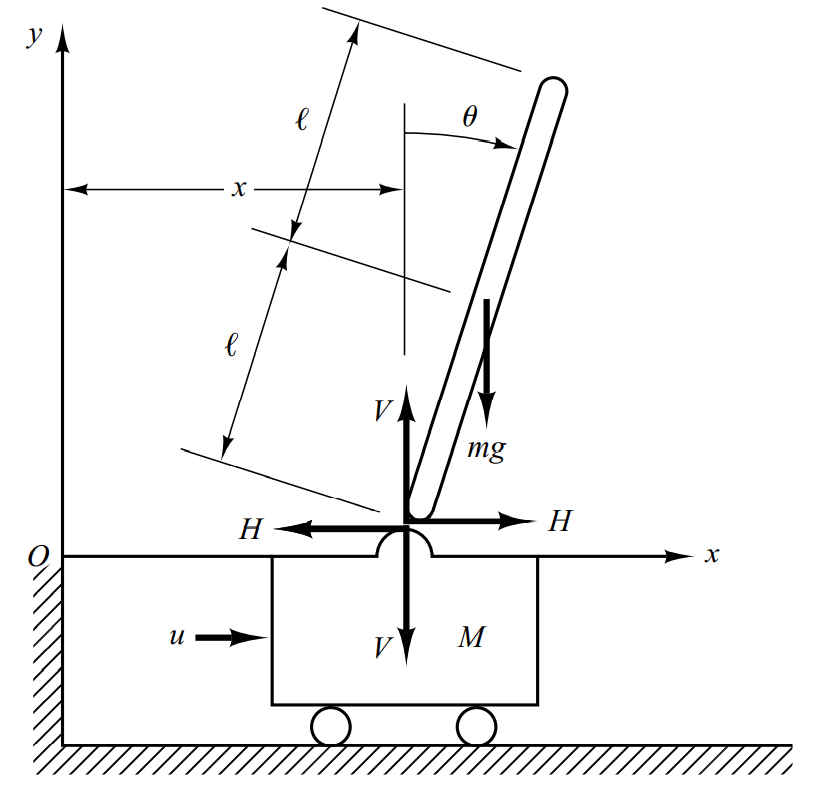如图所示，假设 u u 为外部作用力， M M 为小车质量， m m 为摆杆质量，摆杆长度为 2 l 2l 且摆杆质量均匀分布。设 x x 为小车位置， θ \theta 为摆杆与竖直方向的夹角。系统不考虑空气阻力的影响。

本文给出这些参数如表所示，假设杆的质量是均匀分布的，并且不考虑系统的摩擦。

物理量数值
小车质量( M M ) 2 k g 2kg
摆杆质量( m m ) 0.1 k g 0.1kg
摆杆长度( 2 l 2l ) 1 m 1m

## 1.1.牛顿运动定律分析

先对小车进行受力分析，水平方向有 M x ¨ + H = u \begin{aligned} M\ddot{x}+H = u \end{aligned}

再对摆进行受力分析，水平方向有 H = m d 2 d t 2 ( x + l sin ⁡ θ ) \begin{aligned} H = m\dfrac{d^2}{dt^2}(x+l\sin\theta) \end{aligned}

H = m x ¨ + m l ( θ ¨ cos ⁡ θ − θ ˙ 2 sin ⁡ θ ) \begin{aligned} H = m\ddot{x}+ml(\ddot{\theta}\cos{\theta}-\dot{\theta}^2\sin{\theta}) \end{aligned}

摆的竖直方向有 V − m g = m d 2 d t 2 ( l cos ⁡ θ ) \begin{aligned} V -mg = m\dfrac{d^2}{dt^2}(l\cos{\theta}) \end{aligned}

V = m g − m l ( θ ¨ sin ⁡ θ + θ ˙ 2 cos ⁡ θ ) \begin{aligned} V = mg -ml(\ddot{\theta}\sin{\theta} + \dot{\theta}^2\cos\theta) \end{aligned}

对摆应用动量矩定理，以 θ \theta 顺时针方向旋转为正，设摆绕着质心的转动惯量为 I I ，则有
V l sin ⁡ θ − H l cos ⁡ θ = I θ ¨ \begin{aligned} Vl\sin\theta - Hl\cos\theta = I \ddot{\theta} \end{aligned}

整理可得 ( M + m ) x ¨ + m l θ ¨ cos ⁡ θ − m l θ ˙ 2 sin ⁡ θ = u m l x ¨ cos ⁡ θ + ( I + m l 2 ) θ ¨ − m g l sin ⁡ θ = 0 \begin{aligned} \begin{array}{l} (M+m) \ddot{x}+m l \ddot{\theta}\cos \theta -m l\dot{\theta}^{2} \sin \theta =u \\ m l\ddot{x} \cos \theta +(I+m l^{2}) \ddot{\theta}-m g l \sin \theta=0 \\ \end{array}\end{aligned}

写成矩阵形式如下： [ M + m m l cos ⁡ θ m l cos ⁡ θ I + m l 2 ] [ x ¨ θ ¨ ] + [ 0 − m l θ ˙ sin ⁡ θ 0 0 ] [ x ˙ θ ˙ ] = ( u m g l sin ⁡ θ ) \begin{aligned} {\left[\begin{array}{cc} M+m & m l \cos \theta \\ m l \cos \theta & I+m l^{2} \end{array}\right]\left[\begin{array}{c} \ddot{x} \\ \ddot{\theta} \end{array}\right]+\left[\begin{array}{cc} 0 & -m l \dot{\theta}\sin \theta \\ 0 & 0 \end{array}\right]\left[\begin{array}{l} \dot{x} \\ \dot{\theta} \end{array}\right]=\left(\begin{array}{c} u \\ m g l \sin \theta \end{array}\right)} \end{aligned}

## 1.2.欧拉-拉格朗日方程分析

欧拉-拉格朗日方程可以描述为 d d t ( ∂ T ∂ q ˙ ) − ∂ T ∂ q + ∂ V ∂ q = F \begin{aligned} \dfrac{d}{dt}\left( \dfrac{\partial T}{\partial\dot q}\right) - \dfrac{\partial T}{\partial q} + \dfrac{\partial V}{\partial q} = F \end{aligned} 其中 q q 为系统的广义坐标系， F F 为系统的广义力, T T 为系统动能， V V 为系统势能。

则对于一阶倒立摆系统来说，选择小车位移 x x 和倒立摆的角度 θ \theta 作为广义坐标，小车推力 u u 为广义力，则有
T = 1 2 M x ˙ 2 + 1 2 I θ ˙ 2 + 1 2 m { [ d d t ( x + l sin ⁡ θ ) ] 2 + [ d d t ( l cos ⁡ θ ) ] } V = m g l cos ⁡ θ \begin{aligned} \begin{array}{l} T=\frac{1}{2} M \dot{x}^{2}+\frac{1}{2} I \dot{\theta}^{2}+\frac{1}{2} m\left\{\left[\frac{d}{d t}(x+l \sin \theta)\right]^{2}+\left[\frac{d}{d t}(l \cos \theta)\right]\right\}\\ V=mgl \cos \theta \end{array}\end{aligned}

计算欧拉-拉格朗日方程中的各项，有 d d t ( ∂ T ∂ x ˙ ) = ( M + m ) x ¨ + m l cos ⁡ θ θ ¨ − m l sin ⁡ θ θ ˙ 2 ∂ T ∂ x = 0 , ∂ V ∂ x = 0 d d t ( ∂ T ∂ θ ˙ ) = I θ ¨ + m l ( x ¨ cos ⁡ θ − x ˙ sin ⁡ θ θ ˙ + l θ ¨ ) ∂ T ∂ θ = − m l x ˙ θ ˙ sin ⁡ θ , ∂ V ∂ θ = − m g l sin ⁡ θ , \begin{aligned} \begin{array}{l} \dfrac{d}{d t}\left(\dfrac{\partial T}{\partial \dot{x}}\right)=(M+m) \ddot{x}+m l \cos \theta \ddot{\theta}-m l \sin \theta \dot{\theta}^{2} \\ \dfrac{\partial T}{\partial x}=0, \dfrac{\partial V}{\partial x}=0 \\ \frac{d}{d t}\left(\frac{\partial T}{\partial\dot{\theta}}\right)= I\ddot{\theta}+ml(\ddot{x}\cos{\theta} - \dot{x}\sin{\theta}\dot{\theta}+l\ddot{\theta}) \\ \dfrac{\partial T}{\partial \theta} = -ml\dot{x}\dot{\theta}\sin{\theta}, \dfrac{\partial V}{\partial \theta} = -mgl\sin \theta, \end{array}\end{aligned}

带入欧拉-拉格朗日方程整理可得 ( M + m ) x ¨ + m l θ ¨ cos ⁡ θ − m l θ ˙ 2 sin ⁡ θ = u m l x ¨ cos ⁡ θ + ( I + m l 2 ) θ ¨ + m g l sin ⁡ θ = 0 \begin{aligned} \begin{array}{l} (M+m) \ddot{x}+m l \ddot{\theta}\cos \theta -m l\dot{\theta}^{2} \sin \theta =u \\ m l\ddot{x} \cos \theta +(I+m l^{2}) \ddot{\theta}+m g l \sin \theta=0 \\ \end{array}\end{aligned}

[ M + m m l cos ⁡ θ m l cos ⁡ θ I + m l 2 ] [ x ¨ θ ¨ ] + [ 0 − m l sin ⁡ θ θ ˙ 0 0 ] [ x ˙ θ ˙ ] = [ u m g l sin ⁡ θ ] \begin{aligned} \left[\begin{array}{cc} M+m & m l \cos \theta \\ m l \cos \theta & I+m l^{2} \end{array}\right]\left[\begin{array}{c} \ddot{x} \\ \ddot{\theta} \end{array}\right]+\left[\begin{array}{cc} 0 & -m l \sin \theta \dot{\theta} \\ 0 & 0 \end{array}\right]\left[\begin{array}{c} \dot{x} \\ \dot{\theta} \end{array}\right]=\left[\begin{array}{c} u \\ m g l \sin \theta \end{array}\right] \end{aligned}

可见使用欧拉-拉格朗日方程建立的系统动力学方程与使用牛顿运动定律分析得到的系统动力学方程相同，因此初步验证了所建立的系统动态方程的正确性。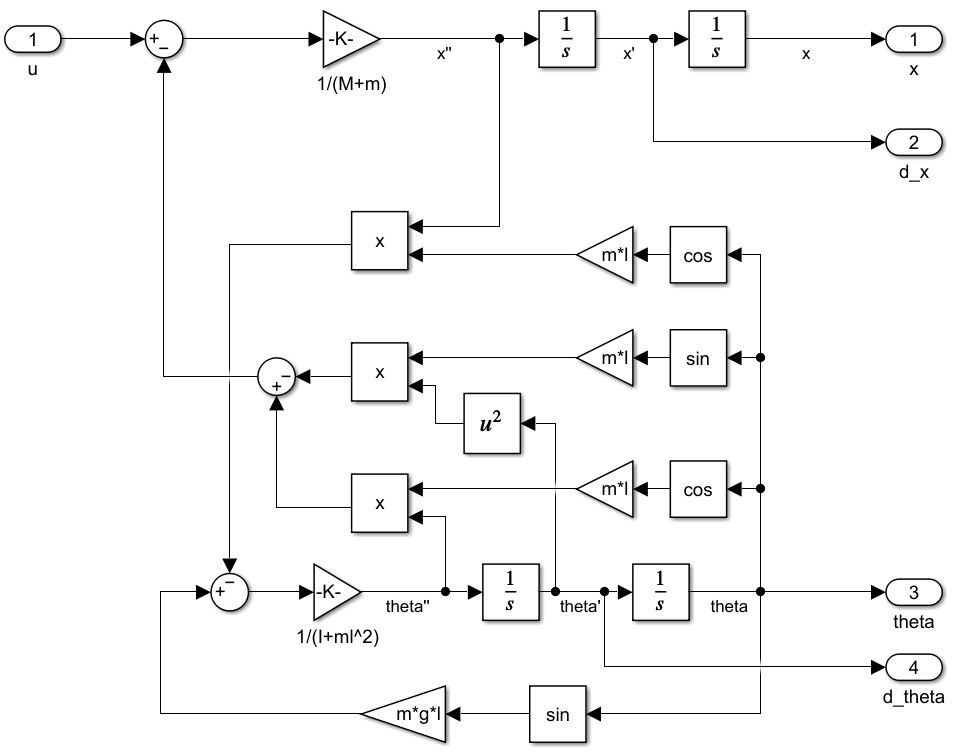假设系统的初始状态为 x = 0 , θ = π 6 x=0,\theta=\frac{\pi}{6} ，且系统无输入，则此后小车位置 x x 和倒立摆的摆角 θ \theta 的变化如图所示。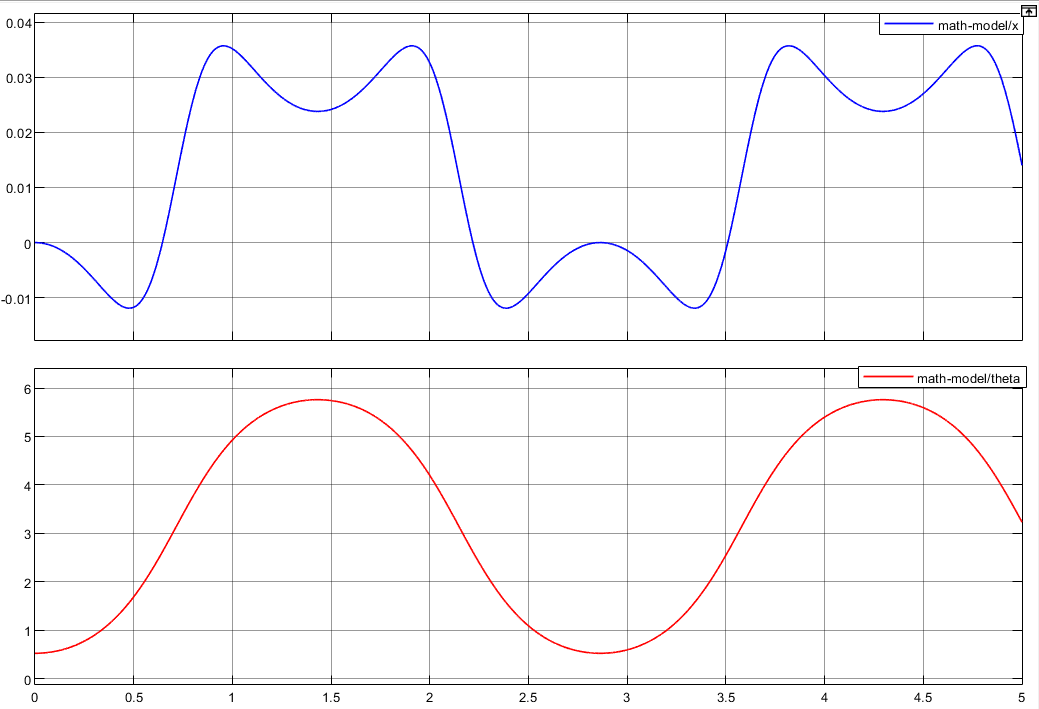# 3.使用SimMechancis仿真

使用SimMechanics可以直接搭建物理模型，只需要对物理模型进行参数赋值和关节的连接就可以完成一个仿真物理模型的搭建。因此这里可以使用SimMechanics建立物理模型进行仿真，并和上面动力学建模的仿真结果进行对比，从而验证上面推导的动力学方程的正确性。如图所示，是使用SimMechanicalcs建立的物理模型。其中增益模块的增益值-1是由于SimMechanicalcs中的 θ \theta 方向和上面推导的方向相反。如图所示，是使用SimMechanicalcs的仿真结果。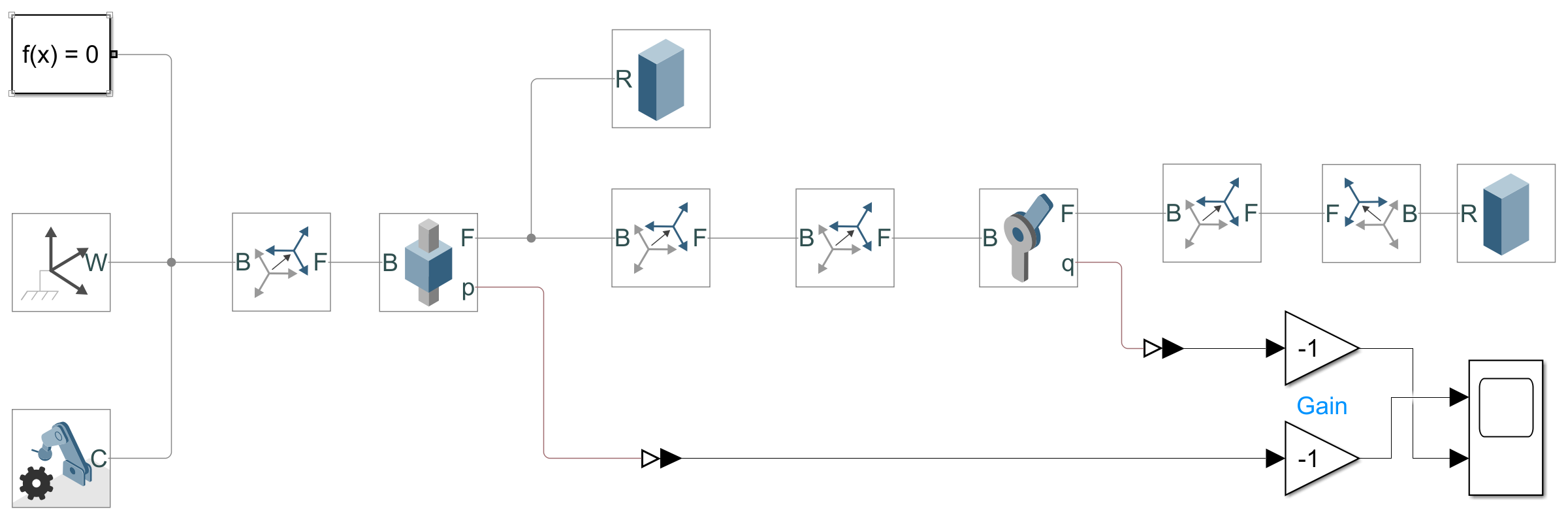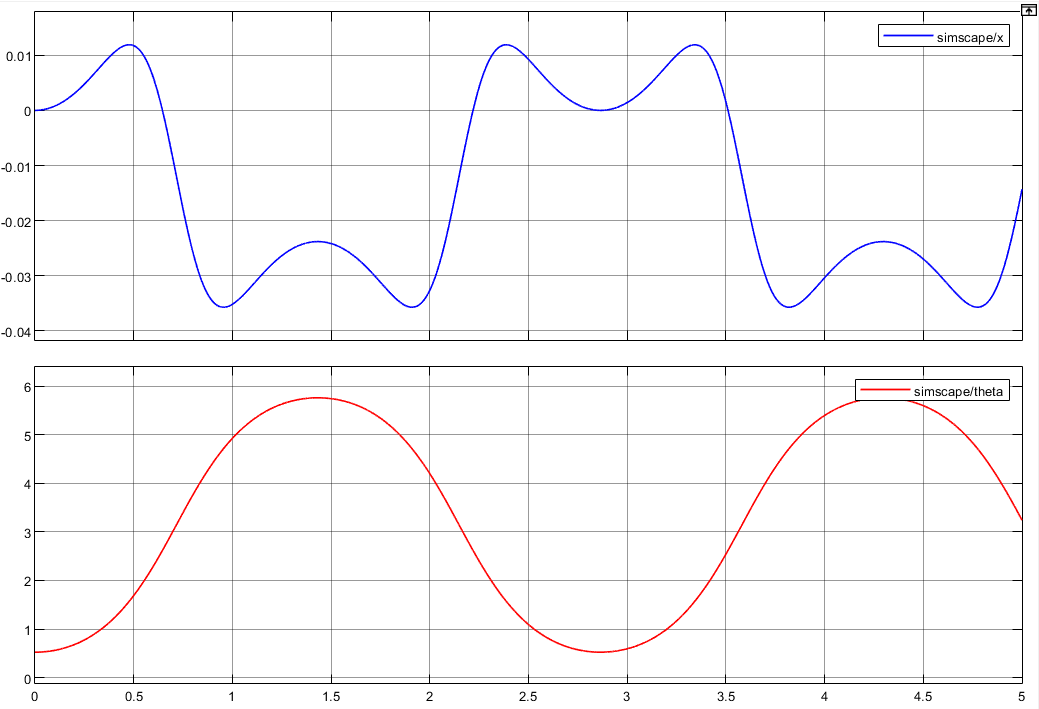# 4.在平衡点附近模型线性化

为了使用线性系统理论的知识对系统进行分析和控制，需要对上述的非线性系统在在平衡点附近进行线性化。系统平衡点附近时， θ \theta 很小，并且假设其角速度 θ ˙ \dot{\theta} 也很小，则可进行近似处理： cos ⁡ θ ≈ 1 , sin ⁡ θ ≈ θ , sin ⁡ θ θ ˙ ≈ 0 \cos{\theta} \approx 1, \sin\theta \approx \theta, \sin \theta \dot{\theta} \approx0 。从而得到一阶倒立摆系统在平衡点附近的线性化模型为
[ M + m m l m l I + m l 2 ] [ x ¨ θ ¨ ] = [ u m g l θ ] \begin{aligned} \left[\begin{array}{cc} M+m & m l \\ m l & I+m l^{2} \end{array}\right]\left[\begin{array}{c} \ddot{x} \\ \ddot{\theta} \end{array}\right]=\left[\begin{array}{c} u \\ m g l \theta \end{array}\right] \end{aligned}

定义系统的状态变量为 ( x 1 , x 2 , x 3 , x 4 ) = ( x , x ˙ , θ , θ ˙ ) (x_1,x_2,x_3,x_4)=(x,\dot{x},\theta,\dot{\theta}) ，系统的输入量为小车外力 u u ，系统输出为小车的位移 x x ，则可得系统的状态空间方程为
[ x ˙ x ¨ θ ˙ θ ¨ ] = [ 0 1 0 0 0 0 − m 2 g l 2 I ( M + m ) + M m l 2 0 0 0 0 1 0 0 m g l ( M + m ) I ( M + m ) + M m l 2 0 ] [ x x ˙ θ θ ˙ ] + [ 0 I + m l 2 I ( M + m ) + M m l 2 0 − m l I ( M + m ) + M m l 2 ] u y = x = [ 1 0 0 0 ] [ x x ˙ θ θ ˙ ] \begin{aligned} \begin{array}{c} {\left[\begin{array}{c} \dot{x} \\ \ddot{x} \\ \dot{\theta} \\ \ddot{\theta} \end{array}\right]=\left[\begin{array}{cccc} 0 & 1 & 0 & 0 \\ 0 & 0 & \dfrac{-m^{2} g l^{2}}{I(M+m)+M m l^{2}} & 0 \\ 0 & 0 & 0 & 1 \\ 0 & 0 & \dfrac{m g l(M+m)}{I(M+m)+M m l^{2}} & 0 \end{array}\right]\left[\begin{array}{c} x \\ \dot{x} \\ \theta \\ \dot{\theta} \end{array}\right]+\left[\begin{array}{c} 0 \\ \dfrac{I+m l^{2}}{I(M+m)+M m l^{2}} \\ 0 \\ \dfrac{-m l}{I(M+m)+M m l^{2}} \end{array}\right] u} \\ y=x=\left[\begin{array}{llll} 1 & 0 & 0 & 0 \end{array}\right]\left[\begin{array}{c} x \\ \dot{x} \\ \theta \\ \dot{\theta} \end{array}\right] \end{array}\end{aligned}

带入系统参数，可得一阶倒立摆系统的状态空间方程为 [ x ˙ x ¨ θ ˙ θ ¨ ] = [ 0 1 0 0 0 0 − 0.3630 0 0 0 1 0 0 15.2444 0 ] [ x x ˙ θ θ ˙ ] + [ 0 0.4938 0 − 0.7407 ] u y = [ 1 0 0 0 ] [ x x ˙ θ θ ˙ ] \begin{aligned} \begin{array}{c} {\left[\begin{array}{c} \dot{x} \\ \ddot{x} \\ \dot{\theta} \\ \ddot{\theta} \end{array}\right]=\left[\begin{array}{cccc} 0 & 1 & 0 & 0 \\ 0 & 0 & -0.3630 \\ 0 & 0 & 0 & 1 \\ 0 & 0 & 15.2444 & 0 \end{array}\right]\left[\begin{array}{c} x \\ \dot{x} \\ \theta \\ \dot{\theta} \end{array}\right]+\left[\begin{array}{c} 0 \\ 0.4938 \\ 0 \\ -0.7407 \end{array}\right] u} \\ y=\left[\begin{array}{llll} 1 & 0 & 0 & 0 \end{array}\right]\left[\begin{array}{c} x \\ \dot{x} \\ \theta \\ \dot{\theta} \end{array}\right] \end{array} \end{aligned}

# 5.系统能控性、能观性和稳定性分析

## 5.1.能控性分析

对于一个连续的时间系统： x ˙ = A x + B u y = C x + D u \begin{aligned} \begin{matrix} \dot{x}=Ax+Bu \\ y= Cx+Du \end{matrix}\end{aligned}

系统能控的充要条件是系统的能控性矩阵为行满秩阵，即 r a n k ( S c ) = r a n k ( [ B A B A 2 B … A n − 1 B ] ) = n \begin{aligned} rank(S_c) = rank(\begin{bmatrix} B & AB & A^2B & \dots & A^{n-1}B \end{bmatrix})=n\end{aligned}

根据上面可控性的原理，对系统进行可控性分析，将式中的 A , B A,B 代入判据中，编写MATLAB代码如下：

M = 2;
m = 0.1;
g = 9.8;
l =0.5;
I = 1/3*m*l^2;

a23 = -m*m*g*l*l/(I*(m+M)+M*m*l*l);
a43 = m*g*l*(M+m)/(I*(m+M)+M*m*l*l);
b2 = (I+m*l*l)/(I*(m+M)+M*m*l*l);
b4 = -m*l/(I*(m+M)+M*m*l*l);

A = [0 1 0 0;
0 0 a23 0;
0 0 0 1;
0 0 a43 0];
B = [0;
b2;
0;
b4];
C =[1 0 0 0];

S_c = [B A*B A^2*B A^3*B]
fprintf('rank(S_c) = %d\n', rank(S_c));


程序运行结果为： r a n k ( S c ) = 4 \begin{aligned} rank(S_c)=4\end{aligned}

因此系统是状态完全可控的。

## 5.2.能观性分析

系统能观的充要条件是系统的能观性矩阵为列满秩阵，即 r a n k ( Q o ) = r a n k ( [ C C A C A 2 ⋮ C A n − 1 ] ) = n \begin{aligned} rank(Q_o) = rank(\begin{bmatrix} C \\C A \\ CA^2 \\ \vdots \\ CA^{n-1} \end{bmatrix})=n\end{aligned}

根据上面能观性原理，继续编写MATLAB代码判断系统能观性

Q_o = [C;
C*A;
C*A^2;
C*A^3];
fprintf('rank(Q_o) = %d\n', rank(Q_o));


程序运行结果为： r a n k ( Q o ) = 4 \begin{aligned} rank(Q_o)=4\end{aligned}

因此系统是能观的。

## 5.3.稳定性分析

### 5.3.1.Routh-Hurwitz判据

劳斯–赫尔维茨稳定性判据给出线性定常系统 ς ( A , B , C ) \varsigma(A,B,C) 输出稳定的充要条件是其传递函数
Φ = C ( s I − A ) − 1 B \begin{aligned} \varPhi = C(sI-A)^{-1}B\end{aligned}
的所有极点都位于复平面S的左半平面，即系统特征方程 ∣ λ I − A ∣ = 0 \mid \lambda I - A\mid = 0 的特征根具有负实部。
使用Matlab计算矩阵A的特征值：

disp('eig(A)=');
eig(A)


程序输出结果为 λ = [ 0 , 0 , 3.9044 , − 3.9044 ] \lambda = \left[0, 0, 3.9044, -3.9044\right] ，存在复平面右半平面的特征根，因此系统是不稳定的。

### 5.3.2.Lyapunov函数

Lyapunov定理给出线性时不变系统在平衡点 x e = 0 x_e = 0 处渐进稳定的充分必要条件是对任意给定的对称正定矩阵 Q Q ，存在一个对称正定矩阵 P P ，满足李雅普诺夫方程 A ⊤ P + P A = − Q \begin{aligned} A^\top P + PA = -Q \end{aligned}

使用MATLAB进行Lyapunov方程的求解，程序如下：

P = lyap(A',eye(4))


程序运行结果为 λ = 1.0 e + 33 × [ − 0.0000 , − 0.0000 , 0.0000 , 3.0550 ] \lambda = 1.0e+33\times\left[-0.0000, -0.0000,0.0000, 3.0550\right] 。由于存在数值稳定性问题仍然解得 P P ，但是 P P 不是正定矩阵，因此系统不稳定。（这里应该有点问题）

# 6.基于极点配置方法的控制器设计

根据上述分析可知系统存在复平面右半平面的极点导致系统不稳定，因此需要设计一个状态反馈控制器 u = − K x u=-Kx ，配置系统闭环极点全部到复平面左半平面。

假设希望将系统的闭环极点配置到 μ 1 = − 1 , μ 2 = − 2 , μ 3 = − 1 + j 3 , μ 4 = − 1 − j 3 \mu_1=-1, \mu_2=-2, \mu_3=-1+j\sqrt{3}, \mu_4=-1-j\sqrt{3} 。则可以通过调用MATLAB中的acker和place命令来方便地计算反馈矩阵K，代码如下：

% 调节器的极点配置问题
J = [-1 -2 -1+j*sqrt(3) -1-j*sqrt(3)];
K1 = acker(A,B,J);
K2 = place(A,B,J);
disp('K1 = ');
disp(K1);
disp('K2 = ');
disp(K2);


程序输出结果为 K 1 = K 2 = ( − 1.1020 , − 2.2041 , − 37.5147 , − 8.2194 ) K1 = K2 = (-1.1020, -2.2041, -37.5147, -8.2194) ，可见对于单输入系统来说使用acker和place命令得到的结果是一样的。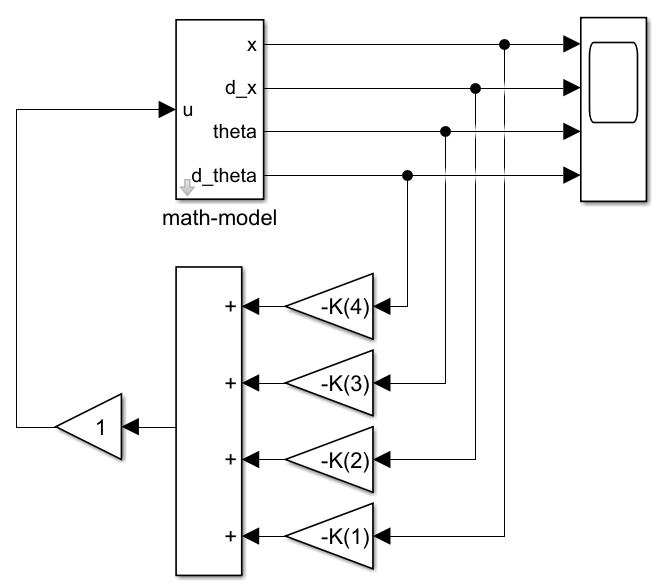假设系统初始状态为 [ x 1 , x 2 , x 3 , x 4 ] = [ x , x ˙ , θ , θ ˙ ] = [ 0 , 0 , 5 ∘ , 0 ] \left[x_1,x_2,x_3,x_4\right]=\left[x,\dot{x},\theta,\dot{\theta}\right]=\left[0,0,5^{\circ},0\right] ，运行Simulink仿真模型，得到系统的闭环响应应如图所示，由图可见系统在微小扰动的情况下仍然能够保持稳定，说明所设计的状态反馈控制器是有效的。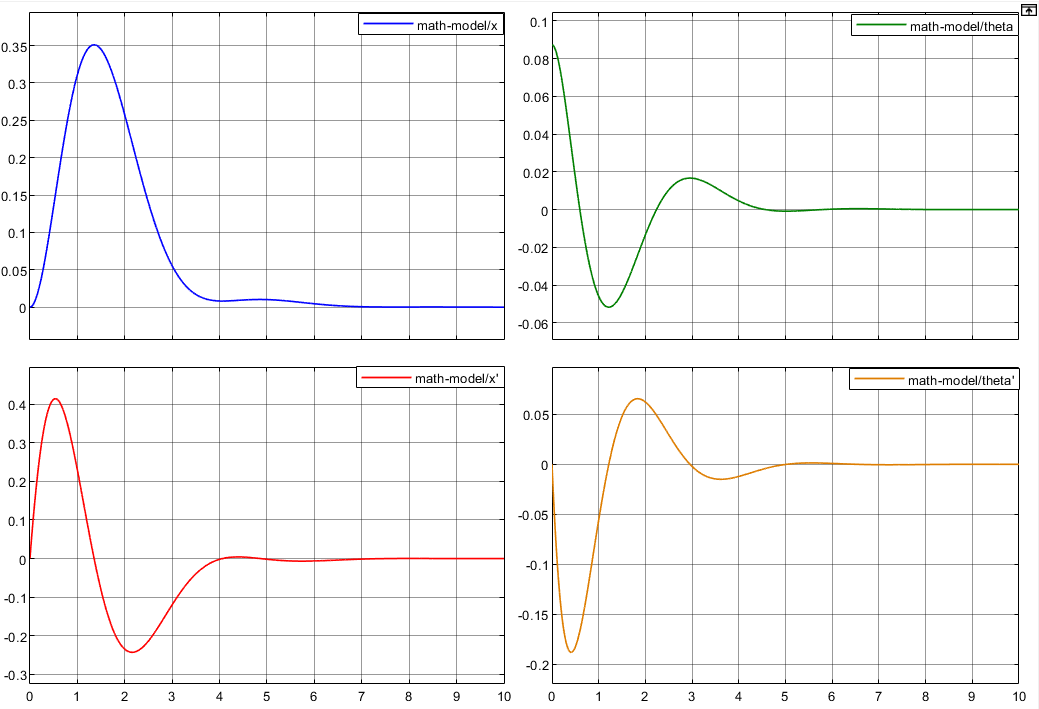# 7.状态观测器设计

## 7.1.全阶观测器

定义线性系统的观测器的数学模型为
x ~ ˙ = ( A − K e C ) x ~ + B u + K e y \begin{aligned} \dot{\widetilde{x}} = (A-K_eC)\widetilde{x}+Bu+K_ey \end{aligned}
其中， x ~ \widetilde{x} 为估计状态， C x ~ C\widetilde{x} 为估计输出。对于观测器的极点配置，一般来说其响应速度至少要比所考虑的闭环系统快2至5倍。因此假设所配置的观测器极点为 μ 1 = − 2 , μ 2 = − 6 , μ 3 = − 2 − j 2 3 , μ 4 = − 2 + j 2 3 \mu_1 = -2, \mu_2 = -6, \mu_3 = -2-j2\sqrt{3}, \mu_4 = -2+j2\sqrt{3}

前文已经验证，取系统的输出变量为 x x ，系统是能观的。因此这里可以直接进行观测器的配置。考虑原系统的对偶系统，可以证明原系统的对偶系统和全解观测器的极点配置问题是等价的，因此可以使用状态反馈极点配置的方法进行极点配置，MATLAB代码如下：

A_ = A';
B_ = C';
C_ = B';
J_ = [-2 -6 -2-j*2*sqrt(3) -2+j*2*sqrt(3)];
Ke = (acker(A_, B_, J_))';
disp('Ke = ');
disp(Ke);


程序输出结果为 K e = ( 12.0 , 75.2 , − 988.9 , − 3689.2 ) Ke = (12.0, 75.2, -988.9, -3689.2)

## 7.2.最小阶观测器

最小阶观测器由全阶观测器中经过变量替换得到，可由下式描述：
η ~ ˙ = A ^ η ~ + B ^ y + F ^ u \begin{aligned} \dot{\tilde{\eta}}=\hat{\boldsymbol{A}} \tilde{\boldsymbol{\eta}}+\hat{\boldsymbol{B}} y+\hat{\boldsymbol{F}} \boldsymbol{u}\end{aligned}
其中， η = x b − K e y η ~ = x ~ b − K e y A ^ = A b b − K e A a b B ^ = A ^ K e + A b a − K e A a a F ^ = B b − K e B a \begin{aligned} \begin{array}{l} \boldsymbol{\eta}= \boldsymbol{x}_{\mathrm{b}}-\boldsymbol{K_e} y\\ \tilde{\boldsymbol{\eta}}=\tilde{\boldsymbol{x}}_{\mathrm{b}}-\boldsymbol{K_e} y\\ \hat{\boldsymbol{A}}=\boldsymbol{A}_{\mathrm{bb}}-\boldsymbol{K_e} \boldsymbol{A}_{\mathrm{ab}} \\ \hat{\boldsymbol{B}} = \hat{\boldsymbol{A}} \boldsymbol{K_e}+\boldsymbol{A}_{\mathrm{ba}}-\boldsymbol{K_e} A_{\mathrm{aa}}\\ \hat{\boldsymbol{F}}= \boldsymbol{B}_{\mathrm{b}}-\boldsymbol{K_e} \boldsymbol{B}_{\mathrm{a}} \end{array}\end{aligned}
则针对 A ^ \hat{\boldsymbol{A}} 进行极点配置即可。

对于一阶倒立摆系统来说，状态变量 x x 等于输出 y y ，因此可以直接测量，剩余的状态变量这是不可测量的部分。对状态空间模型划分如下：
x = [ x a x b ] = [ x x ˙ θ θ ˙ ] , A = [ 0 1 0 0 0 0 − 0.3630 0 0 0 0 1 0 0 15.2444 0 ] , B = [ 0 0.4938 0 − 0.7407 ] \begin{aligned} x = \left[ \begin{array}{c} x_a \\ \hdashline \boldsymbol {x_b} \end{array}\right] = \left[ \begin{array}{c} x \\ \hdashline \dot{x} \\ \theta \\ \dot{\theta} \end{array} \right] , A = \left[\begin{array}{c:ccc} 0 & 1 & 0 & 0 \\ \hdashline 0 & 0 & -0.3630 &0\\ 0 & 0 & 0 & 1 \\ 0 & 0 & 15.2444 & 0 \end{array}\right] , B = \left[ \begin{array}{c} 0 \\ \hdashline 0.4938 \\ 0 \\ -0.7407 \end{array} \right]\end{aligned}

划分后的降阶观测器是3阶系统，假设期望配置的极点位置为 μ 1 = − 6 , μ 2 = − 2 − j 2 3 , μ 3 = − 2 + j 2 3 \mu_1 = -6, \mu_2 = -2-j2\sqrt{3}, \mu_3 = -2+j2\sqrt{3} ，则可编写MATLAB代码如下：
编写代码：

Aaa = A(1, 1);
Aab = A(1, 2:end);
Aba = A(2:end, 1);
Abb = A(2:end, 2:end);
J_j = [-6 -2+j*2*sqrt(3) -2-j*2*sqrt(3)];
Ke_j = (acker(Abb',Aab',J_j))';
disp('Ke_j = ');
disp(Ke_j);


程序输出为 K e j = ( 10 , − 152.2041 , − 684.4898 ) Ke_j = (10, -152.2041, -684.4898)

# 8.基于观测器的状态反馈控制

## 8.1.基于全阶观测器的状态反馈控制

对于线性系统来说，基于全阶观测器的状态反馈控制系统的状态方程为
[ x ˙ e ˙ ] = [ A − B K 0 A − K e C ] [ x e ] \begin{aligned} \begin{bmatrix} \dot{x} \\ \dot{e} \end{bmatrix} = \begin{bmatrix} A & -BK \\ 0 & A-K_eC \end{bmatrix} \begin{bmatrix} x \\ e \end{bmatrix} \end{aligned}

但是这里一阶倒立摆系统是非线性系统，上述观测器和极点配置都是使用线性化的模型得到的，因此式并不能完全真实反映系统状态。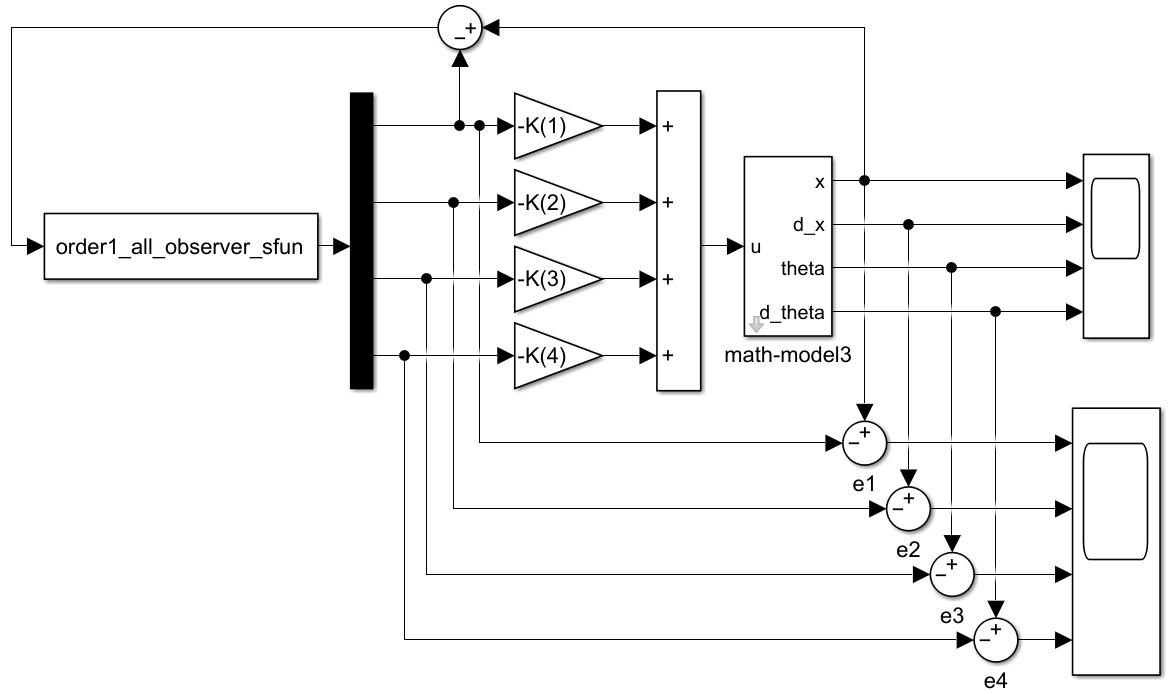% 一阶倒立摆系统的全阶观测器s-function建模
function [sys,x0,str,ts,simStateCompliance]=
order1_all_observer_sfun(t,x,u,flag, x_0, th_0)
switch flag,
case 0,
[sys,x0,str,ts,simStateCompliance]=
mdlInitializeSizes(t,x,u, x_0, th_0);
case 1,
sys=mdlDerivatives(t,x,u);
case 2,
sys=mdlUpdate(t,x,u);
case 3,
sys=mdlOutputs(t,x,u);
case 4,
sys=mdlGetTimeOfNextVarHit(t,x,u);
case 9,
sys=mdlTerminate(t,x,u);
otherwise
num2str(flag));
end
% 主函数结束

% ---------------------------------------------
function [sys,x0,str,ts,simStateCompliance]=
mdlInitializeSizes(t,x,u,x_0, th_0)
% 初始化
sizes = simsizes;% 生成sizes数据结构

sizes.NumContStates  = 4;% 连续状态数, 分别是x', theta1', theta2', x, theta1, theta2
sizes.NumDiscStates  = 0;% 离散状态数,缺省为 0
sizes.NumOutputs     = 4;% 输出量个数,缺省为 0,
sizes.NumInputs      = 1;% 输入量个数，缺省为 0, 这里的输入取为e
sizes.DirFeedthrough = 1;% 是否存在直接馈通。1：存在；0：不存在，缺省为 1
sizes.NumSampleTimes = 1;   % at least one sample time is needed

sys = simsizes(sizes);
x0  = [x_0; 0; th_0; 0];% 设置初始状态
str = [];% 保留变量置空
ts  = [0 0]; % 连续系统
simStateCompliance = 'UnknownSimState';
% end mdlInitializeSizes

% ---------------------------------------------
function sys=mdlDerivatives(t, x, u)
%  计算导数例程子函数
M = 2; m = 0.1; l =0.5; I = 1/3*m*l^2; g = 9.8;
a23 = -m*m*g*l*l/(I*(m+M)+M*m*l*l);
a43 = m*g*l*(M+m)/(I*(m+M)+M*m*l*l);
b2 = (I+m*l*l)/(I*(m+M)+M*m*l*l);
b4 = -m*l/(I*(m+M)+M*m*l*l);
A = [0 1 0 0;
0 0 a23 0;
0 0 0 1;
0 0 a43 0];
b = [0; b2; 0; b4];
K = [-1.1020, -2.2041, -37.5147, -8.2194];
Ke = [12; 75.2; -988.9; -3689.2];
sys = (A-b*K)*x + Ke*u ;  % 注意这里的u就是x(1)的观测误差e(1)

% ---------------------------------------------
function sys=mdlUpdate(t,x,u)
%3. 状态更新例程子函数
sys = [];

% ---------------------------------------------
function sys=mdlOutputs(t,x,u)
%4. 计算输出例程子函数
sys=x;

% ---------------------------------------------
function sys=mdlGetTimeOfNextVarHit(t,x,u)
% 5. 计算下一个采样时间，仅在系统是变采样时间系统时调用
sampleTime = 1;    %  Example, set the next hit to be one second later.
sys = t + sampleTime;

% ---------------------------------------------
function sys=mdlTerminate(t,x,u)
% 6. 仿真结束时要调用的例程函数
sys = [];


假设系统初始状态为 [ x 1 , x 2 , x 3 , x 4 ] = [ x , x ˙ , θ , θ ˙ ] = [ 0 , 0 , 5 ∘ , 0 ] \left[x_1,x_2,x_3,x_4\right]=\left[x,\dot{x},\theta,\dot{\theta}\right]=\left[0,0,5^{\circ},0\right] ，并且状态观测器的初始观测误差为 [ e 1 , e 2 , e 3 , e 4 ] = [ 0 , 0 , 5 ∘ , 0 ] \left[e_1,e_2,e_3,e_4\right]=\left[0,0,5^{\circ},0\right] ，运行Simulink仿真模型，系统的动态响应如图所示，全阶观测器的观测误差如图，可见系统可以很好地稳定。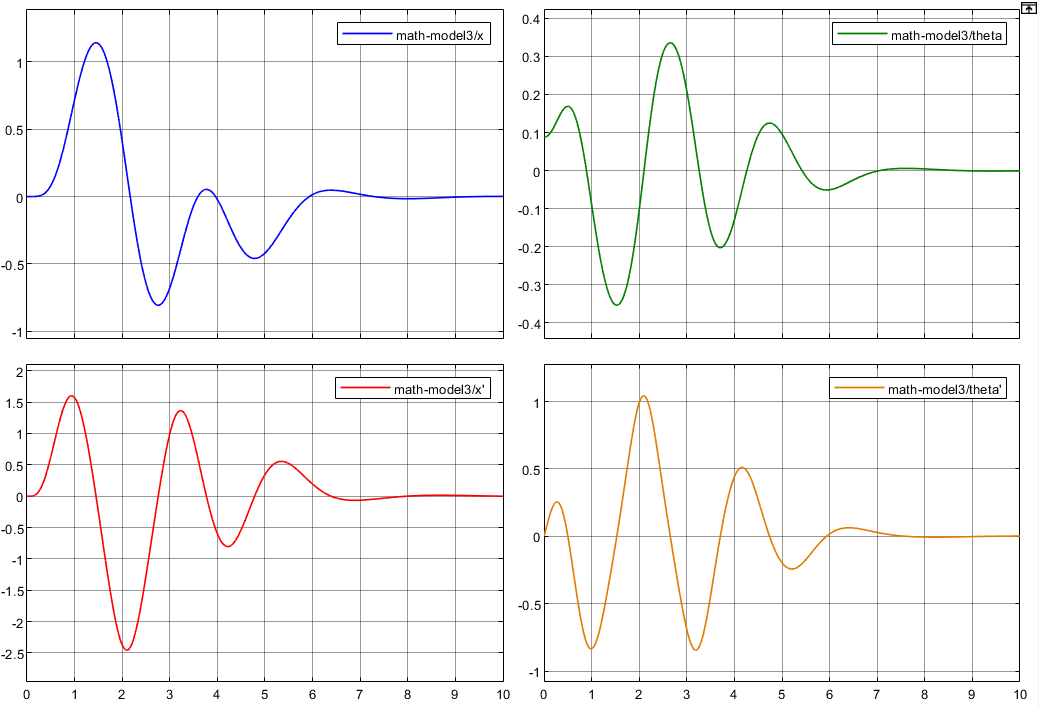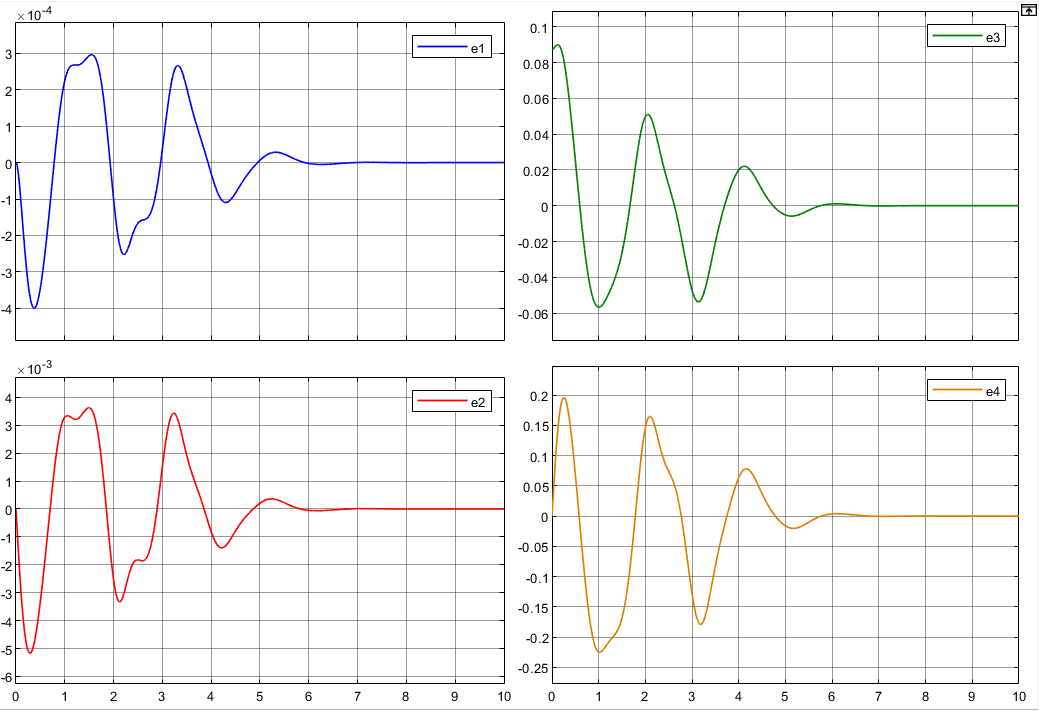## 8.2.基于最小观测器的状态反馈控制

对于线性系统来说，基于最小阶观测器的状态反馈控制系统的状态方程为
[ x ˙ e ˙ ] = [ A − B K B K b 0 A b b − K e A a b ] [ x e ] \begin{aligned} \begin{bmatrix} \dot x \\ \dot{e} \end{bmatrix} = \begin{bmatrix} A - BK & BK_b \\ 0 & A_{bb} - K_eA_{ab} \end{bmatrix} \begin{bmatrix} x \\ e \end{bmatrix}\end{aligned}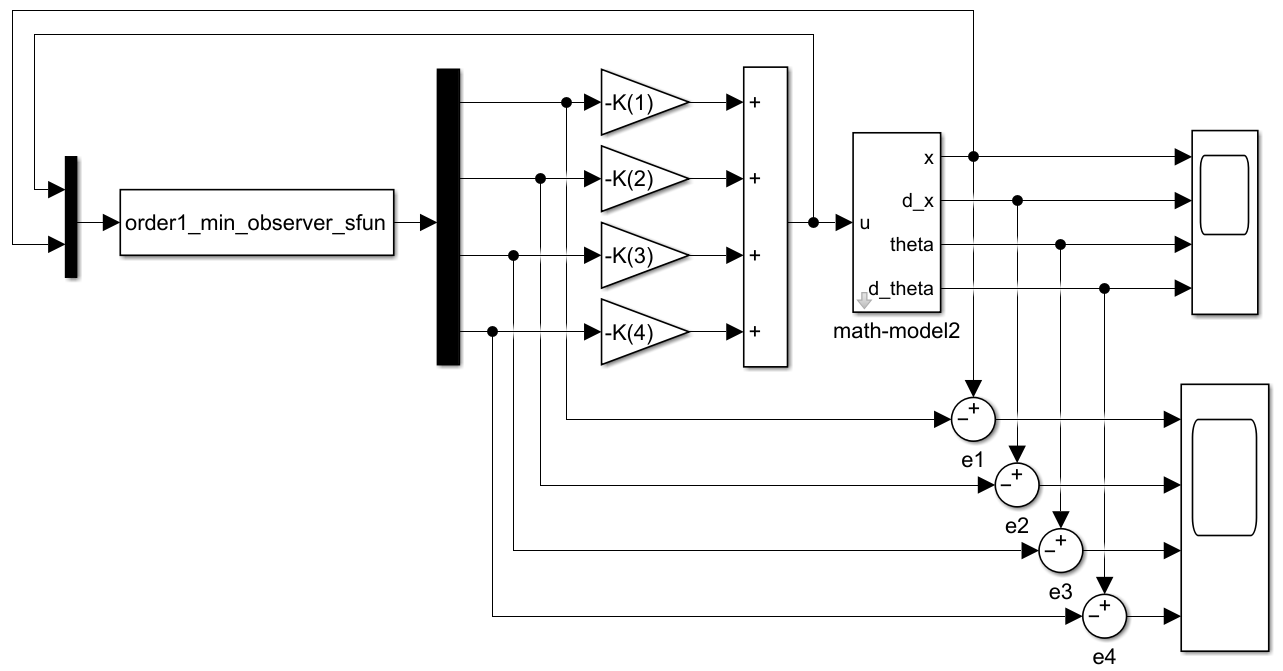% 一阶倒立摆系统的最小阶观测器s-function建模
function [sys,x0,str,ts,simStateCompliance] =
order1_min_observer_sfun(t,x,u,flag, x_0, th_0)
switch flag,
case 0,
[sys,x0,str,ts,simStateCompliance]=
mdlInitializeSizes(t,x,u, x_0, th_0);
case 1,
sys=mdlDerivatives(t,x,u);
case 2,
sys=mdlUpdate(t,x,u);
case 3,
sys=mdlOutputs(t,x,u);
case 4,
sys=mdlGetTimeOfNextVarHit(t,x,u);
case 9,
sys=mdlTerminate(t,x,u);
otherwise
num2str(flag));
end
% 主函数结束

% ---------------------------------------------
function [sys,x0,str,ts,simStateCompliance]=
mdlInitializeSizes(t,x,u,x_0, th_0)
% 初始化
sizes = simsizes;% 生成sizes数据结构

sizes.NumContStates  = 3;% 连续状态数
sizes.NumDiscStates  = 0;% 离散状态数,缺省为 0
sizes.NumOutputs     = 4;% 输出量个数,缺省为 0,
sizes.NumInputs      = 2;% 输入量个数，缺省为 0, 这里的输入取为输入u和y
sizes.DirFeedthrough = 1;% 是否存在直接馈通。1：存在；0：不存在，缺省为 1
sizes.NumSampleTimes = 1;   % at least one sample time is needed
sys = simsizes(sizes);
x0  = [0; th_0; 0] - [10; -152.2041; -684.4898]*x_0;  % 设置初始状态
str = [];% 保留变量置空
ts  = [0 0]; % 连续系统
simStateCompliance = 'UnknownSimState';
% end mdlInitializeSizes

% ---------------------------------------------
function sys=mdlDerivatives(t, x, u)
%  计算导数例程子函数
M = 2; m = 0.1; l =0.5; I = 1/3*m*l^2; g = 9.8;
a23 = -m*m*g*l*l/(I*(m+M)+M*m*l*l);
a43 = m*g*l*(M+m)/(I*(m+M)+M*m*l*l);
b2 = (I+m*l*l)/(I*(m+M)+M*m*l*l);
b4 = -m*l/(I*(m+M)+M*m*l*l);
A = [0 1 0   0;
0 0 a23 0;
0 0 0   1;
0 0 a43 0];
B = [0; b2; 0; b4];
Ke_j = [10;  -152.2041; -684.4898];
A_hat = A(2:end, 2:end) - Ke_j*A(1, 2:end);
B_hat = A_hat*Ke_j + A(2:end, 1) - Ke_j*A(1,1);
F_hat = B(2:end) - Ke_j*B(1);
sys = A_hat*x + B_hat*u(2) + F_hat*u(1) ;  % u1就是输入，u2是原系统输出y

% ---------------------------------------------
function sys=mdlUpdate(t,x,u)
%3. 状态更新例程子函数
sys = [];

% ---------------------------------------------
function sys=mdlOutputs(t,x,u)
%4. 计算输出例程子函数
C_hat = [0 0 0; 1 0 0; 0 1 0; 0 0 1];
D_hat = [1; 10; -152.2041; -684.4898];
sys = C_hat*x + D_hat*u(2);

% ---------------------------------------------
function sys=mdlGetTimeOfNextVarHit(t,x,u)
% 5. 计算下一个采样时间，仅在系统是变采样时间系统时调用
sampleTime = 1;    %  Example, set the next hit to be one second later.
sys = t + sampleTime;

% ---------------------------------------------
function sys=mdlTerminate(t,x,u)
% 6. 仿真结束时要调用的例程函数
sys = [];


假设系统初始状态为 [ x 1 , x 2 , x 3 , x 4 ] = [ x , x ˙ , θ , θ ˙ ] = [ 0 , 0 , 5 ∘ , 0 ] \left[x_1,x_2,x_3,x_4\right]=\left[x,\dot{x},\theta,\dot{\theta}\right]=\left[0,0,5^{\circ},0\right] ，并且最小阶状态观测器的初始观测误差为 [ e 2 , e 3 , e 4 ] = [ 0 , 5 ∘ , 0 ] \left[e_2,e_3,e_4\right]=\left[0,5^{\circ},0\right] 。运行Simulink仿真模型，系统的动态响应如图所示，最小阶观测器的观测误差如图，其中由于状态变量 x 1 x_1 是小车的位移，即系统输出可以直接测量，因此它的误差一直是0。可见基于最小阶观测器的系统动态响应速度比使用全阶观测器效果更好，这是因为有一个状态变量可以进行准确的观测，因此系统收敛速度更快。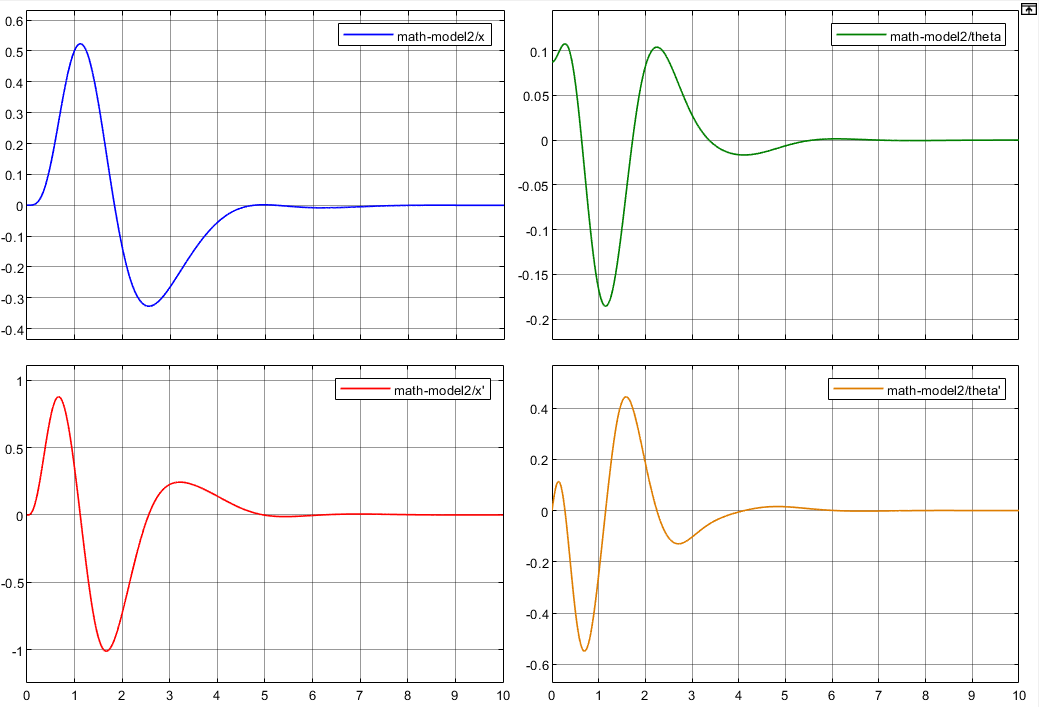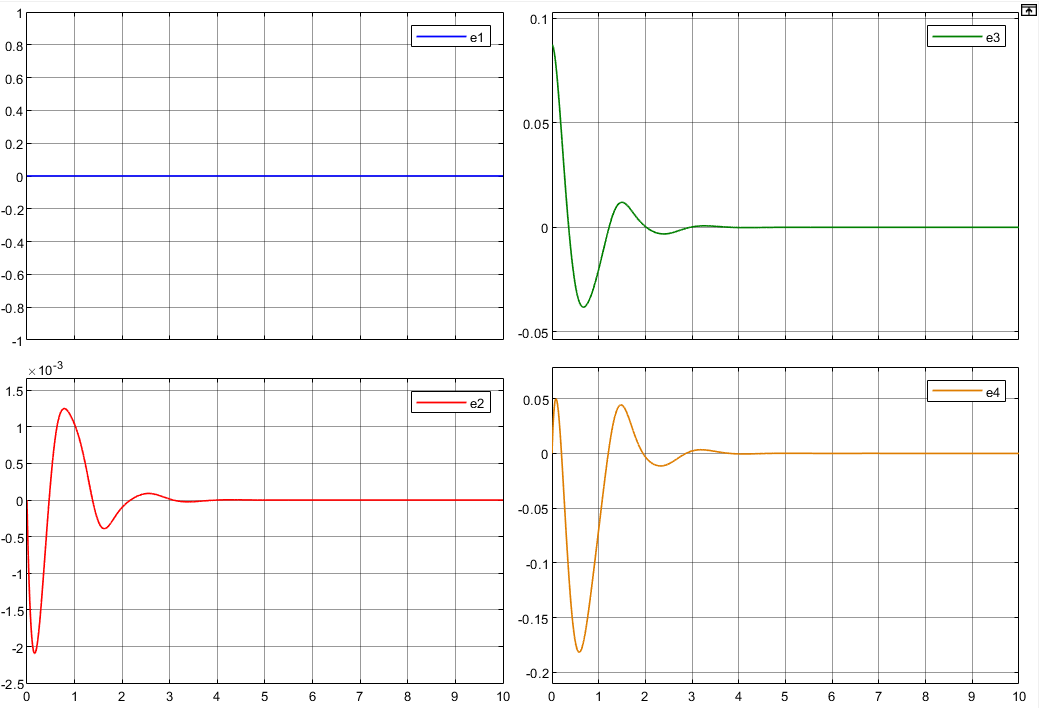# 9.LQR控制

已知系统的状态空间模型 x ˙ = A x + B u \begin{aligned} \dot{x} &= Ax+Bu \end{aligned}
LQR问题就是确定下列最佳控制向量的矩阵 K K u ( t ) = − K x ( t ) \begin{aligned} u(t)=-Kx(t)\end{aligned} 使得下列性能指标达到最小值：
J = ∫ 0 ∞ [ x ⊤ Q x + u ⊤ R u ] d t \begin{aligned} J = \int_0^\infty [\boldsymbol x^\top\boldsymbol{Qx} + \boldsymbol u^\top \boldsymbol{Ru}]dt\end{aligned}

选择 Q = I , R = I \boldsymbol Q= \boldsymbol I,\boldsymbol R= \boldsymbol I ，使用MATLAB求解的代码如下：

Q = eye(4);
R = eye(1);
K_lqr = lqr(A,B,Q,R);
disp('K_lqr = ');
disp(K_lqr);


得到最优状态反馈矩阵为 K l q r = ( − 1 , − 2.7964 , − 53.9967 , − 13.9239 ) K_lqr = (-1, -2.7964, -53.9967, -13.9239)

直接使用这个反馈控制矩阵在Simulink中仿真非线性模型的LQR控制，假设系统初始状态为 [ x 1 , x 2 , x 3 , x 4 ] = [ x , x ˙ , θ , θ ˙ ] = [ 0 , 0 , 5 ∘ , 0 ] \left[x_1,x_2,x_3,x_4\right]=\left[x,\dot{x},\theta,\dot{\theta}\right]=\left[0,0,5^{\circ},0\right] ，系统的动态响应如图所示，可见系统的控制效果非常好。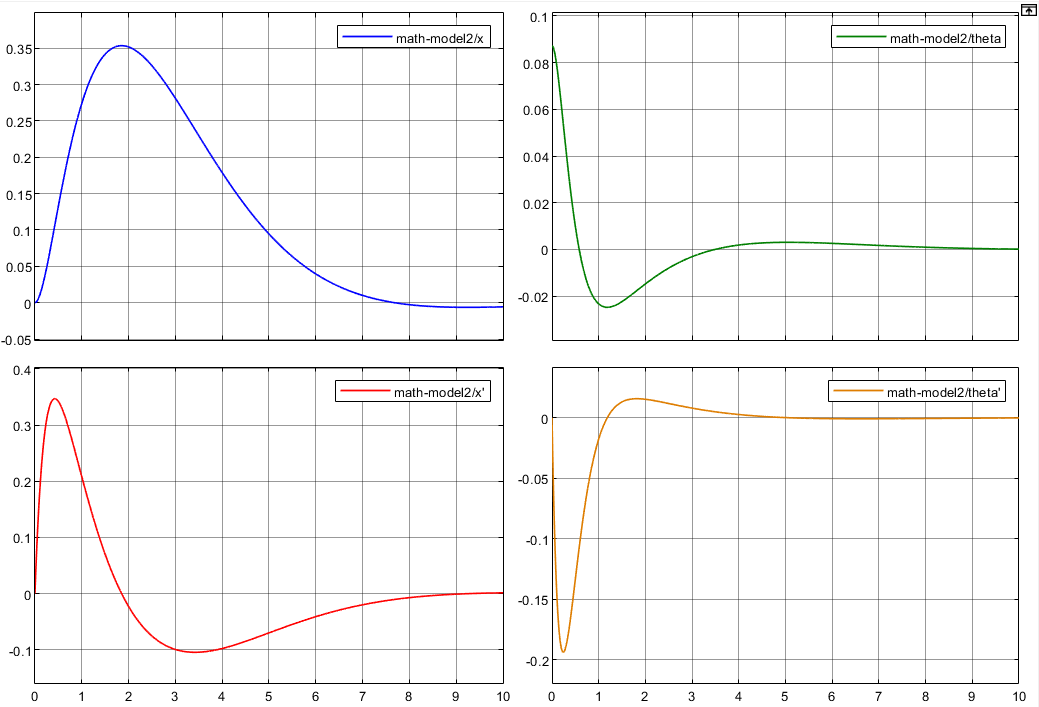展开全文控制器
• 基于固高公司的倒立摆设备，一阶倒立摆的PID控制，包括建模及仿真，PID控制算法
• 三、直线型一阶倒立摆模型建立 一级倒立摆系统是一个不稳定的系统，需要对其进行机理建模。 在研究过程中，应忽略空气摩擦、等，而后可将倒立摆系统进行抽象化，认为其由小车和匀质刚性杆两部分组成并对这两部分...

### 三、直线型一阶倒立摆模型建立

一级倒立摆系统是一个不稳定的系统，需要对其进行机理建模。 在研究过程中，应忽略空气摩擦、等，而后可将倒立摆系统进行抽象化，认为其由小车和匀质刚性杆两部分组成并对这两部分进行如图所示的受力分析: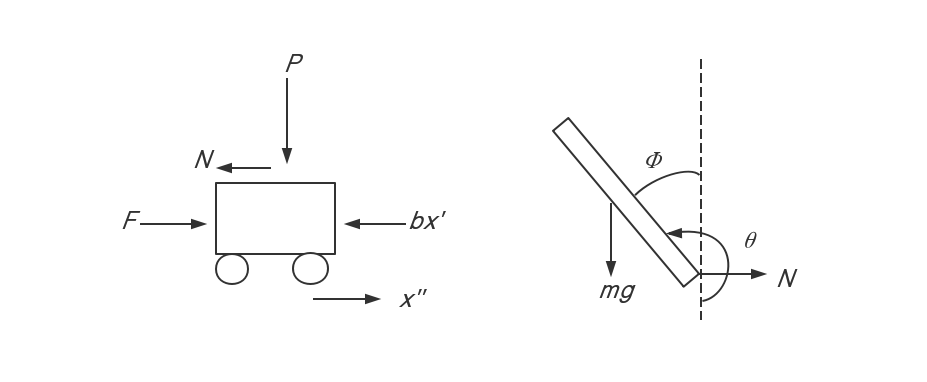其中为小车的质量和摆杆的质量；b、Fx分别为小车的摩擦系数、施加在小车上的作用力和小车的位置；I和 分别为摆杆的惯量和摆杆转动轴心到质心的长度； 和  分别为摆杆与竖直向上方向和竖直向下方向的夹角；N P 分别为摆杆作用力的水平与竖直分量。

小车水平方向的合力：

(1)

摆杆水平方向的合力:

(2)

摆杆水平方向运动方程：

(3)

摆杆力矩平衡方程：

(4)

摆杆在竖直方向的合力：

(5)

可得到摆杆在竖直方向的运动方程：

(6)

摆杆竖直方向运动方程：

(7)

将作用力 F 用 u代替,同时进行线性化,即得到:

(8)

(9)

其中为小角度。

质量均匀分布的摆杆，对于式(8)有                                                          (11)

由式(8)、(11)得到：                                                      (12)

对质量均匀摆杆，取,可得到线性一阶直线倒立摆状态空间描述：

(13)

(14)

对系统进行可控性分析，由控制矩阵Qc=[B  AB    ]=,用matlab计算可知，Qc的秩为4，系统可控。系统可进行状态变量的极点配置。

对系统进行可观测性分析，由观测矩阵Qo=[C  CA    ],用matlab计算可知，Qo的秩为4，系统可观测。系统可设计观测器，并且观测器可控。

### 直线型一阶倒立摆7---总结

有需要直线型一阶倒立摆的VREP仿真文件:可点击

展开全文• 五、能量起摆 能量起摆这一概念来自于K.J.Astrom and K.Furuta的... 摘要：这篇文章介绍了能量控制和展示了如何用该方法设计策略控制倒立摆起摆。 导论 倒立摆起摆是一个经典的控制学实验，see Furutaet...

### 五、能量起摆

能量起摆这一概念来自于K.J.Astrom and K.Furuta的SWINGING UP A PENDULUM BY ENERGY CONTROL。文献下载地址

以下是我翻译的部分论文。

摘要：这篇文章介绍了能量控制和展示了如何用该方法设计策略控制倒立摆起摆。

1. ### 导论

倒立摆起摆是一个经典的控制学实验，see Furutaet al.(1992).Furutaet al的最小时间控制。这个。控制测量并不强健。这篇论文将要介绍能量控制问题。The pendulum is simply controlled in such a way that its energy is driven towards a value equal to the steady·state upright position.使用适当的控制策略可使倒立摆达到正确的位置。分析表明，这个问题完全可用两个参数表达。一个是time scale另一个是摆杆的最大加速与重力加速度之比。这个结论适用广义复杂的倒立摆。

### 2. PRELIMINARIES

考虑单级倒立摆。设它的质量为m，转动惯量关于枢轴点为j，并且设I为从枢轴到质心的距离。垂直和钟摆之间的夹角是θ，其中θ在时钟方向上是正的。重力加速度为g和质心加速度为u。

The equations of motion of the pendulum is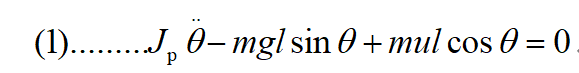系统有两个状态变量，角度θ和角速度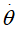。It is natural to let the state space be a cylinder.在这个状态空间中系统有两个平衡点为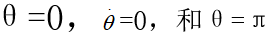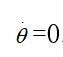方程一基于许多假设，其中摩擦力忽略和假定倒立摆为刚体。同时也假定了轴向速度没有限制。

### 3.ENERGY CONTROL

多次测试表明能量控制倒立摆能够能够替代位置控制和速率，参见Wiklund et al.(1993).For example one way to swing the pendulum to the upright position is to give it an energy that corresponds to the upright position.倒立摆将起摆并在适当位置被稳摆算法控制。Equation (2).为了解决能量控制的有效性，需要理解质心加速度如何影响能量。Computing the deriva-tive of E with respect to time we find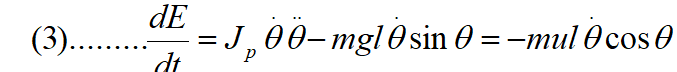由式(3)可知，能量易于控制。该系统只是一个增益变化的积分器。当(3)的右边的u的系数消失时，就失去了可控性。

这发生在0 = 0或0 =±tr/2时，即，当摆是水平的或当它的速度逆转。当角B为0或ff且速度较大时，控制作用最为有效，为增加能量，当B cos{}为负时，主u的加速为正。

### 4.SWING UP STRATEGIES

我们现在要讨论让倒立摆上升到合适位置的方法。先假设倒立摆开始处于竖直稳定状态。倒立摆初始能量为-2mgl。(upright position)合适位置的能量为0.It is very convenient to use argu-ments based on control of the energy of the pendulum.(利用摆的能量控制原理进行论证是非常方便的。一种起摆的方法是控制倒立摆让它的能量从-2mgl增加到0.然后，倒立摆起摆到合适的位置会被稳摆程序捕获，并稳定倒立摆。可以看出，这类策略将收敛到期望的解决方案，见Fradkov(1991)和Frad-kov等(1995)。一个简单的方法就可以实现。假定倒立摆处于竖直位置。朝任意方向输出最大加速度，当速率变为零的时候然后颠倒加速度方向。(Accelerate with max-imum acceleration in an arbitrary direction and re-verse the acceleration when the velocity becomes zero. )为了了解这种策略会发生什么，我们引入一个固定在枢轴点上的坐标系。在这个坐标系中，摆的质心作圆周运动，如图l所示。如果加速度向右，则在OB方向上有一个磁场，其表观重力大小为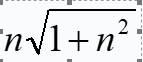in the direction OB.然后钟摆对称地围绕OB摆动。考虑如下，倒立摆在A点时速率为0.倒立摆将摆到位置C角度为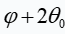，参加图一C点的速率将为0.此，钟摆的摆幅增加了一个相应的角度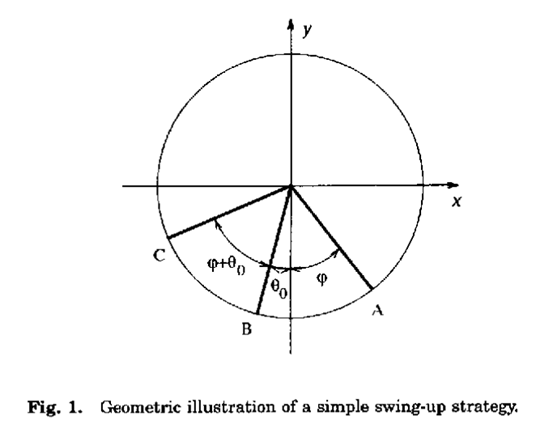为每一个反向的速度。注意，这个简单的策略只有在钟摆没有到达地平线时才是最优的，因为从式(4)可以看出，当钟摆水平时，加速度应该是反向的。还请注意，必须要求它是可能保持最大加速度在充分摆动。

### 5.SINGLE-SWING BEHAVIOR

摆升的行为主要取决于枢轴的最大加速度。如果参数n足够大，就能很快地获得所需的能量，而且摆可以一次摆起来，但如果n很小，则需要多次摆起来。

Double-Switch Control

如果加速度足够的大，在输出最大加速度的情况下倒立摆很容易起摆，直到获得期望能量，再将加速度设置为0.使用这种方法控制信号开关从0变到最大值，再从最大值变为0.这也是该方法命名的缘由。找到这个策略，我们将考虑一个固定在摆的枢轴上的坐标系，并把由于枢轴加速度产生的力看作是一个外力。在这个坐标系中，摆的质心沿半径为I的圆周运动。由式(4)可知，摆达到水平位置前必须达到所需要的能量。如果支点的加速度一直保持在最大值ng，直到达到水平，支点的加速度提供给摆的能量为nmgl。摆上摆的能量必须增加2mgl，因此我们发现最大加速度必须至少是2g。当加速度为2g时，摆被加速到水平，此时控制信号为零。如果加速度大于2g，当摆摆角ф时，加速度将被关闭。提供给摆的能量是nmgl sinф。Equating this with 2mgl gives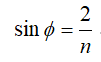。单开关双开关策略如图2所示，它给出了n = 2.1时的角度、角速度和控制信号。注意，在达到所需的能量之前使用最大控制动作。这发生在钟摆水平之前。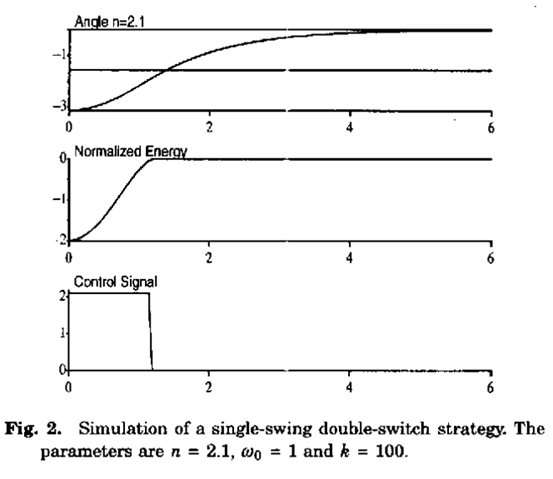Large Accelerations

如果可用加速度远远大于2g，就有可能找到开关时间的近似表达式。我们将考虑钟摆从向下的位置开始时的情况。

如果加速度如此之大，以致于在小角度下可以得到所需的能量，则运动方程(1)可以近似为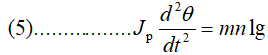这个方程的解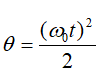。倒立摆能量增加为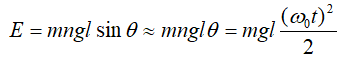。使它等于起摆能量2mgl.求得完全加速时间为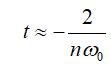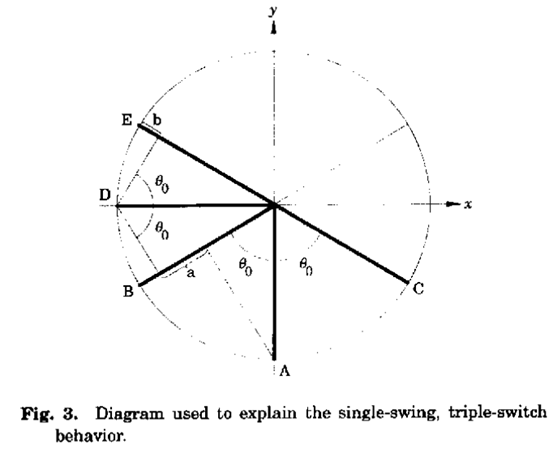其中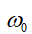为摆小振动的频率，摆改变角度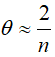比较精确值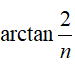)。当n = 10时，我们得到e.g。在加速过程中，给定的加速度让倒立摆摆动了11.5°。

Triple-Switch Control

在上面讨论的单摆策略中，摆向一边并继续摆，直到摆到垂直位置。即使最大加速度小于2g，也有可能找到钟摆以同方式运动的策略。然而，控制信号会切换几次。加速度必须大于g，才能使摆在一次摆动中达到水平。“磅找出更大的是我们将考虑情况如图3所示,钟摆开始在其他位置,最大加速度ng首次应用于积极的方向,一个观察者固定在主蜜蜂的引力场OB方向的能量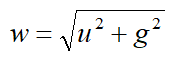当摆锤从A移动到D时，它失去势能mwa = mw（sinθ -cosθ），它被转换为动能，从公式（3）得出，枢轴的控制加速度应该反转当摆锤是水平的，以便最大化摆锤的能量。当加速度反转时，固定在枢轴上的观察者看到OC方向上的重力场，摆锤的动能必须足够大才能带来摆到位置E.势能的差异是mwb，因此我们得到条件a> b。从图3可以得出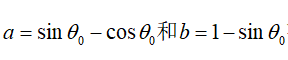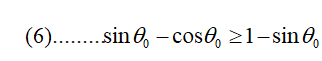引入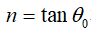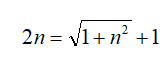该等式具有解n = 4/3。因此，加速度必须至少为4g/3才能具有单摆行为。当加速度恰好等于4g /3时，枢轴将首先向右加速，直到摆锤到达水平位置。然后反转加速直到摆锤达到所需的能量，当a，'celeration设置为零时，当n大于4/3时，摆锤的加速度可以在摆锤到达点K之前设置为零当枢轴的加速度设定为零时，让摆锤与水平面形成角度ф。提供给摆的能量则为mgl（2-sin ф），因为枢轴通过加速度移动距离1+ L（l-sinф），等于2mgl给出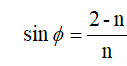For n = 4/3 we get ф = 30' .

单摆三开关控制如图4所示。最初施加最大控制信号。能量增加但是当摆锤是水平时它没有达到期望的水平。 'lb继续向钟摆供电，然后反转控制信号，直到获得所需的能量。然后将控制信号设置为零。由于对于这种类型的行为，n = 2.1接近极限n = 2，当控制信号设置为零时，摆锤接近期望的直立位置。

### 6.MULTI-SWING BEHAVIOR

如果最大加速度小于4g / 3，则需要在摆锤到达直立位置之前多次摆动摆锤。让我们首先考虑将摆锤放在图5所示的两个摆动中的条件，图5显示了固定在枢轴上的坐标系。该坐标系中的观察者看到强度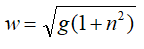。如果枢轴的加速度为正，则磁场具有方向OB，而当磁极为负时，该磁场具有方向OC。假设摆锤在A处静止时开始，并且首先在正方向上给出最大加速度。然后钟摆在图中从A摆动到C.此时摆锤转动，枢轴的加速度反转。当摆锤在E处到达水平位置时，枢轴的加速度再次反转。如果E处的速度足够大，则钟摆将达到直立位置。钟摆在D处休息时。当它移动到E时，它已经失去了潜在的能量mwa，它已被转移到动能。这个动能必须足够大才能将摆锤移动到F.这个能量是mwb，我们得到条件b≥a。从图5可以看出a = sin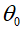-cos 3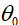和b = 1 -sin因此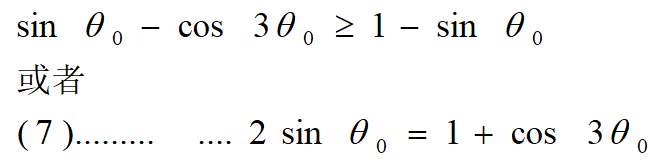该等式具有解决方案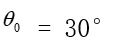。因此所需的最大加速度为0.58g。控制信号在该策略中切换四次，因为必须在D处反向控制以减小摆的能量。

The General Case

很容易将论证扩展到需要更多波动的情况。例如，在具有三次摆动的策略中，钟摆首先在一个方向上摆动2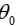。下一次向另一个方向摆动4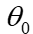，到达直立位置的状态变为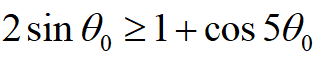A case with k swings gives the equation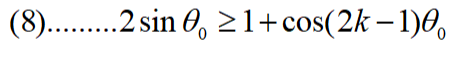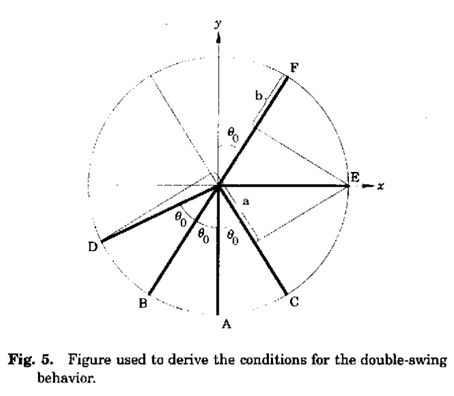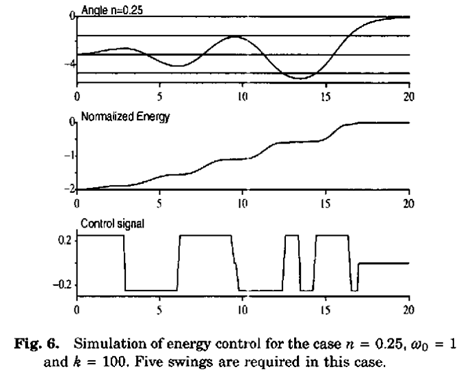以数字方式求解该等式，我们得到以下值：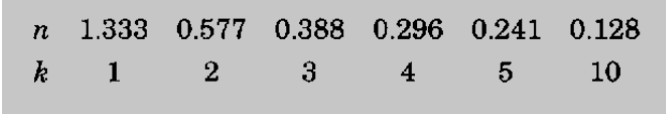对于n的小值，n和k之间的关系近似由下式给出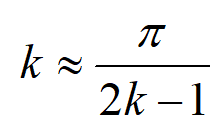所需的开关数量随着n的减小而增加。当n = 0.25时，我们发现需要五个开关来摆动摆锤。这在图6中示出了图示。

7.MINIMUM TIME STRATEGIES

从Pontryagins最大原则可以看出，摆锤摆动的最小时间策略是bang-bang型。可以证明，这些策略可以很好地解释为能量控制。它们将以最大速率向钟摆注入能量，然后以最大速率移除能量，使得当达到直立位置时能量对应于平衡能量。对于n的小值，最小时间策略给出的控制信号与最初基于能量控制的策略相同。然而，控制信号的最后部分是不同的，因为我们已经描述的策略将在获得所需能量时将控制信号设置为零。因此，所给出的策略可以被描述为能量中没有过冲的策略。

考虑例如情况n> 2，其中可以使用单摆动策略。 10摆动pendu-lum它的能量必须增加2mgl。这可以通过Fignre 2中所示的单摆双开关策略来实现。最大加速度一直使用，直到摆锤移动角度arctan2 / n。通过继续加速可以进一步增加能量，直到摆锤到达水平位置。从方程（3）得出，加速度应该相反。通过将加速度反转到适当的位置，可以减小能量，使得当摆锤处于水平时它达到所需的值。通过计算细节，我们发现角度获得了最大能量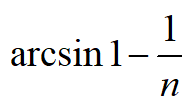然后以最大速率降低能量。图7比较了最小时间策略和能量控制策略。该图还显示，到达直立位置的时间差异随着n的增加而增加。它还表明最小时间策略的能量过冲。过冲随着n的增加而增加。对于n = 5的情况，过冲超过200％。这就解释了为什么最小时间策略非常敏感。当钟摆接近右上位置时，多余的能量迅速消失。能量控制为直立位置提供了更多的方法。通常结合几种不同的策略来摆动钟摆。当钟摆接近直立位置时使用捕捉策略。一个好的实践方法是使用能量超过10-20％的能量控制策略，并在接近垂直位置时抓住笔杆。这种策略很简单，对于建模工具非常有用。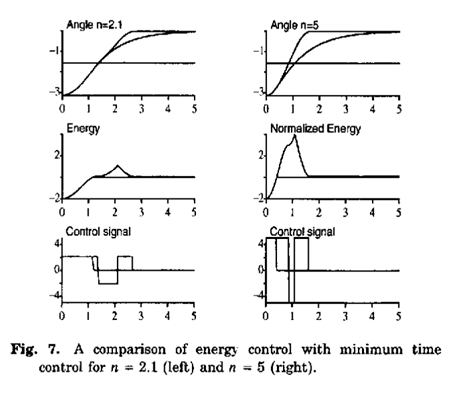### 8.CONCLUSION

已经表明，通过控制其能量来摆动摆锤是非常方便的。由等式（4）给出的控制策略非常简单。使用此策略获得的行为主要取决于一个参数，即可用的加速度。如果加速度足够大，u> 2g，摆锤可以一次摆动到达直立位置。控制信号使用其最大值，直到获得所需的能量，然后设置为零。对于u> 4g / 3，摆锤仍可以一次摆动，但控制信号现在可以制作三个开关。对于较低的加速度，摆锤必须摆动几次。

### 9. 参考文献

K. Furuta, M. Yamakita, and S. Kobayashi (1992), Swing-up control of inverted pendulum using pseudo-state feed-back. J. Systems and Control Eng. 206, pp. 263-269. M. Wiklund, A. KristenBon, and K. J. Astrom (1992, A new strategy for swinging up an inverted pendulum. Proc. IFAC 12th World Congress, Vo\. 9, pp. 151-154. Fradkov, A. L. (1991), Speed-gradient laws of control and evolution. Proc. 1st European Control Conference, Greno-ble, pp. 1861-1865. Fradkov, A. L., P. Y. Guzenko, D. J. Hill, and A. y. Pogromsky (1995), Speed gradient control and passivity of nonlinear oscillators. Proc. IFAC Symposium on Control of Nonlinear Systems, Lake Tahoo.

### 直线型一阶倒立摆7---总结

有需要直线型一阶倒立摆的VREP仿真文件:可点击

展开全文...